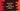# JavaScript string codePointAt method explanation with example## JavaScript string codePointAt method:

The codePointAt is an inbuilt method of JavaScript string. This method is used to get the unicode code point value at a given position of a string.

In this post, we will learn the syntax of codePointAt and how to use codePointAt with examples.

### Syntax of codePointAt:

The syntax of codePointAt is:

``codePointAt(p)``

Where,

• p is the position of an element in the string to get the code point value

It returns a decimal value.

### Return value of codePointAt:

The codePointAt method returns a decimal value that represents the code point of the character at the given position p.

### Edge cases:

Following are the edge cases of codePointAt:

• If there is no element at position p, it returns undefined.
• If the element is UTF-16 high surrogate, it returns the code point of the surrogate pair.
• If the element is UTF-16 low surrogate, it will return code point of the low surrogate.

### Examples of codePointAt:

Let’s learn how codePointAt works with different examples:

``````let givenStr = 'Hello';

console.log(givenStr.codePointAt(0));
console.log(givenStr.codePointAt(1));
console.log(givenStr.codePointAt(2));``````

It will print:

``````72
101
108``````

Let’s print the string value of the code points:

``````let givenStr = 'Hello';

console.log(givenStr.codePointAt(0).toString(16));
console.log(givenStr.codePointAt(1).toString(16));
console.log(givenStr.codePointAt(2).toString(16));``````

These will print the values in hexadecimal:

``````48
65
6c``````

Let’s try this with smileys:

``````let givenStr = '🐟🐳🐋🦈🌾🌎';

console.log(givenStr.codePointAt(0));
console.log(givenStr.codePointAt(1));
console.log(givenStr.codePointAt(2));``````

It will print:

``````128031
56351
128051``````